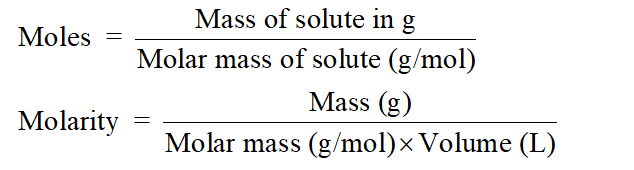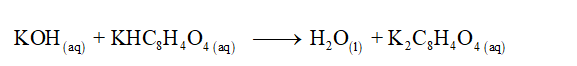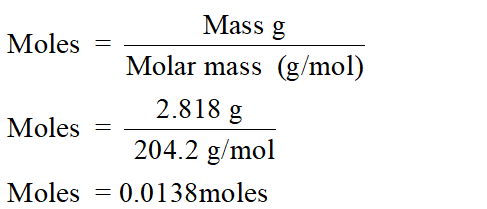# When 2.818 g of potassium hydrogen phthalate (symbolized here as KHPh; molar mass =204.2 g/mol) is titrated with KOH solution, it takes 31.74 mL of the base to achieve the end point. Calculate the concentration of the KOH solution.

Question
1 views

When 2.818 g of potassium hydrogen phthalate (symbolized here as KHPh; molar mass =204.2 g/mol) is titrated with KOH solution, it takes 31.74 mL of the base to achieve the end point. Calculate the concentration of the KOH solution.

check_circle

Step 1

Solution stoichiometry is mainly based on the calculation of moles and volumes. These two values are used to calculate the molarity of solution. The relation between moles, and mass with molarity is as given below;Step 2

The balance chemical reaction between potassium hydrogen phthalate and KOH can be written as:Step 3

Mass of potassium hydrogen phthalate=2.818 g

Molar mass of potassium hydrogen phthalate = 204.2 g/mol

Calculate the moles of potassium hydrogen phthalate:...

### Want to see the full answer?

See Solution

#### Want to see this answer and more?

Solutions are written by subject experts who are available 24/7. Questions are typically answered within 1 hour.*

See Solution
*Response times may vary by subject and question.
Tagged in